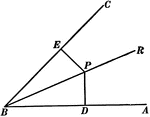### Perpendiculars To The Sides Of An Angle

Illustration used to show "The two perpendiculars to the sides of an angle from any point in its bisector…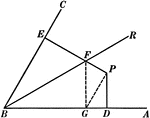### Perpendiculars To The Sides Of An Angle

Illustration used to show "The two perpendiculars to the sides of an angle from any point not in its…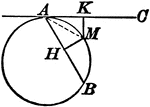### Circle With a Tangent Line and Chord

Illustration showing that from any point in the circumference of a circle, a chord and a tangent are…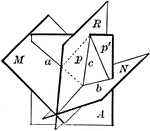### Plane Bisecting Dihedral Angle

Diagram used to prove the theorem: "Every point in a plane which bisects a dihedral angle is equidistant…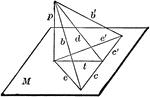### Perpendicular To Line In Plane

"If from the foot of a perpendicular to a plane a straight line is drawn at right angles to any line…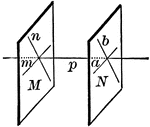### Planes Perpendicular To A Line

"Two planes perpendicular to the same straight line are parallel."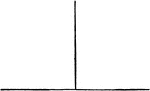### Perpendicular Lines

Illustration of perpendicular lines. Intersecting lines that meet at a right angle.### Perpendicular Lines With Horizontal and Vertical Labeled

Illustration of perpendicular lines with the horizontal and vertical lines labeled. "A horizontal line…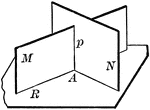### 2 Intersecting Planes Perpendicular To A Third Plane

"If two intersecting planes are each perpendicular to a third plane, their intersection is also perpendicular…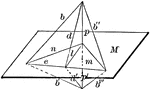### Line Perpendicular To Plane

"If a straight line is perpendicular to each of two straight lines at their point of intersection it…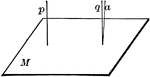### Lines Perpendicular To A Plane

"If one of two parallels is perpendicular to a plane, the other is also."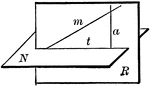### Plane Passed Perpendicular To A Given Plane

"Through a given line oblique to a plane, one, and only one plane, can be passed perpendicular to the…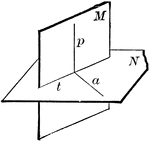### Perpendicular Planes

"If two planes are perpendicular to each other, a line in one of them perpendicular to the intersection…### Perpendicular Between 2 Straight Lines

Diagram used to prove the theorem: "Between two straight lines not in the same plane only one perpendicular…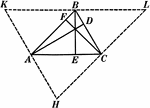### Altitudes In A Triangle

Illustration used to show "The altitudes of a triangle are concurrent."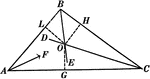### Angle Bisectors In A Triangle

Illustration used to prove "The bisectors of the angles of a triangle are concurrent in a point which…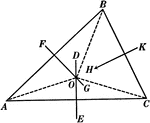### Perpendicular Bisectors In A Triangle

Illustration used to prove "The perpendicular bisectors of the sides of a triangle are concurrent in…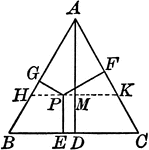### Perpendiculars Dropped Any Point in the Equilateral Triangle

Illustration to show the perpendiculars dropped from any point in the equilateral triangle to the three…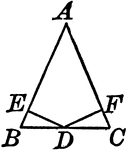### Perpendiculars Dropped From Midpoint of Base to Legs of Isosceles Triangles

Illustration to show the perpendiculars dropped from the midpoint of the base to the legs of an isosceles…